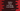# Use any() function in python to check if anything inside a iterable is True## Python any():

An iterable is an object that returns an iterator. An iterator is used to iterate through an object. Examples of iterables in python are list, tuple, string, dictionary etc._ any()_ in python is used to check if any element in an iterable is True. That means it will return True if anything inside an iterable is True, else it will return False. The syntax of any() is as below :

```````any(iterable)
```````

The iterable parameter in the above function is an iterable object, such as list, tuple, set, dictionary or a string. In this tutorial, we will learn how any() behaves with these iterables.

## Example of using any() :

As we have explained earlier, any() will return the following outputs : True: If any value is true. True: If all values are true. True: If any value is false, but not all. False: If all the values are false. False: If the iterable is empty.

### any() with a list :

Python list holds different comma separated values inside a square bracket ([]). Let’s try to use any() with a list :

### Explanation :

As you can see in the example above, any() is useful for checking if an item in a list is ‘False’ or if the list is empty. Instead of iterating through the list to check if all items are ’True’ or not, we can use ‘any()’. Let’s analyze each print statements one by one.

The commented numbers in the above program denote the step number below :

1. Only one value is 0, but others are non-zero. So it results True.
2. All are non-zero numbers. So, True.
3. True because all are non-zero numbers and one value is True.
4. One value is False but others are non-zero numbers. So, the result is True.
5. False because it is an empty list.
6. False because members are either zero or False.

### any() with a tuple :

Tuples are used to hold a sequence of objects in Python. Python tuples are similar to Python lists. They are used to hold a sequence of objects. But, tuples are immutable, unlike lists. We can’t change them. The items are comma separated and placed inside a parenthesis. We can use any() with a tuple similarly like a list.

The output is the same as the above example. Only the last two print statement will print ‘False’.

### any() with string :

A string is also iterable and we can use any() on a string object as well. As you can see that only for the empty string, it results False. For non-empty strings, the result is always True. This is another way to check if a string is empty or not.

### any() with dictionaries :

Dictionaries are a list of key-value pairs. any() will check only the keys,i.e. if any one of the keys is true, it will result True. Else, False

‘fourthdict’ has the first element with key ‘False’ and ‘fifthdict’ is an empty dictionary. The result is ‘False’ for both.

### Conclusion :

You can use any() in any iterable to check quickly if all values are False or not. Try to run the programs on your side and let me know if you have any queries.